# Sphere

For other uses, see Sphere (disambiguation).

A sphere is a symmetrical geometrical object. In non-mathematical usage, the term is used to refer either to a round ball or to its two-dimensional surface. In mathematics, a sphere is the set of all points in three-dimensional space (R3) which are at distance r from a fixed point of that space, where r is a positive real number called the radius of the sphere. Thus, in three dimensions, a mathematical sphere is considered to be a spherical surface, rather than the volume contained within it. The fixed point is called the center, and is not part of the sphere itself. The special case of r = 1 is called a unit sphere.

This article deals with the mathematical concept of a sphere. In physics, a sphere is an object (usually idealized for the sake of simplicity) capable of colliding or stacking with other objects that occupy space.

## Equations in R3

In analytic geometry, a sphere with center (x0, y0, z0) and radius r is the locus of all points (x, y, z) such that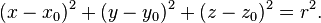$(x - x_0 )^2 + (y - y_0 )^2 + ( z - z_0 )^2 = r^2.$

The points on the sphere with radius r can be parametrized via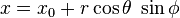$x = x_0 + r \cos \theta \; \sin \phi$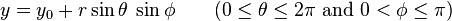$y = y_0 + r \sin \theta \; \sin \phi \qquad (0 \leq \theta \leq 2\pi \mbox{ and } 0 < \phi \leq \pi ) \,$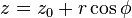$z = z_0 + r \cos \phi \,$

A sphere of any radius centered at the origin is described by the following differential equation: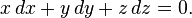$x \, dx + y \, dy + z \, dz = 0.$

This equation reflects the fact that the position and velocity vectors of a point traveling on the sphere are always orthogonal to each other.

The surface area of a sphere of radius r is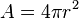$A = 4 \pi r^2 \,$

and its enclosed volume is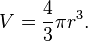$V = \frac{4}{3}\pi r^3.$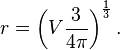$r = \left(V \frac{3}{4\pi}\right)^\frac{1}{3}.$

The sphere has the smallest surface area among all surfaces enclosing a given volume and it encloses the largest volume among all closed surfaces with a given surface area. For this reason, the sphere appears in nature: For instance, bubbles and small water drops are roughly spherical, because the surface tension locally minimizes surface area.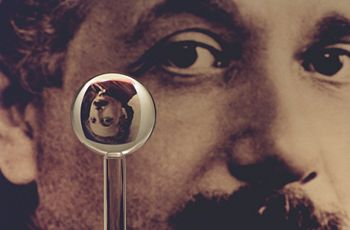An image of one of the most accurate spheres ever created by humans, as it refracts the image of Einstein in the background. This sphere was a fused quartz gyroscope for the Gravity Probe B experiment which differs in shape from a perfect sphere by no more than 40 atoms of thickness. It is thought that only neutron stars are smoother. It was announced on June 15, 2007, that Australian scientists are planning on making even more perfect spheres, accurate to 35 millionths of a millimeter, as part of an international hunt to find a new global standard kilogram.

The circumscribed cylinder for a given sphere has a volume which is 1.5 times the volume of the sphere, and also the curved portion has a surface area which is equal to the surface area of the sphere. This fact, along with the volume and surface formulas given above, was already known to Archimedes.

A sphere can also be defined as the surface formed by rotating a circle about any diameter. If the circle is replaced by an ellipse, and rotated about the major axis, the shape becomes a prolate spheroid, rotated about the minor axis, an oblate spheroid.

## Terminology

Pairs of points on a sphere that lie on a straight line through its center are called antipodal points. A great circle is a circle on the sphere that has the same center and radius as the sphere, and consequently divides it into two equal parts. The shortest distance between two distinct non-antipodal points on the surface and measured along the surface, is on the unique great circle passing through the two points.

If a particular point on a sphere is designated as its north pole, then the corresponding antipodal point is called the south pole, and the equator is the great circle that is equidistant to them. Great circles through the two poles are called lines (or meridians) of longitude, and the line connecting the two poles is called the axis of rotation. Circles on the sphere that are parallel to the equator are lines of latitude. This terminology is also used for astronomical bodies such as the planet Earth, even though it is neither spherical nor even spheroidal.

A sphere is divided into two equal hemispheres by any plane that passes through its center. If two intersecting planes pass through its center, then they will subdivide the sphere into four lunes, or biangles, the vertices of which all coincide with the antipodal points lying on the line of intersection of the planes.

## Generalization to other dimensions

Spheres can be generalized to spaces of any dimension. For any natural number n, an n-sphere, often written as Sn, is the set of points in (n+1)-dimensional Euclidean space which are at a fixed distance r from a central point of that space, where r is, as before, a positive real number. In particular:

• a 0-sphere is a pair of endpoints of an interval (−r, r) of the real line
• a 1-sphere is a circle of radius r
• a 2-sphere is an ordinary sphere
• a 3-sphere is a sphere in 4-dimensional Euclidean space.

Spheres for n > 2 are sometimes called hyperspheres.

The n-sphere of unit radius centered at the origin is denoted Sn and is often referred to as "the" n-sphere. Note that the ordinary sphere is a 2-sphere, because it is a 2-dimensional surface, though it is also a 3-dimensional object because it can be embedded in ordinary 3-space.

The surface area of the (n−1)-sphere of radius 1 is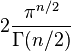$2 \frac{\pi^{n/2}}{\Gamma(n/2)}$

where Γ(z) is Euler's Gamma function.

Another formula for surface area is$\begin{cases} \displaystyle \frac{(2\pi)^{n/2}\,r^{n-1}}{2 \cdot 4 \cdots (n-2)} , & \text{if } n \text{ is even}; \\ \\ \displaystyle \frac{2(2\pi)^{(n-1)/2}\,r^{n-1}}{1 \cdot 3 \cdots (n-2)} , & \text{if } n \text{ is odd}. \end{cases}$

and the volume within is the surface area times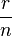${r \over n}$ or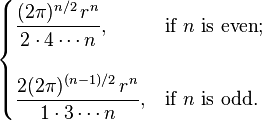$\begin{cases} \displaystyle \frac{(2\pi)^{n/2}\,r^n}{2 \cdot 4 \cdots n} , & \text{if } n \text{ is even}; \\ \\ \displaystyle \frac{2(2\pi)^{(n-1)/2}\,r^n}{1 \cdot 3 \cdots n} , & \text{if } n \text{ is odd}. \end{cases}$

## Generalization to metric spaces

More generally, in a metric space (E, d), the sphere of center x and radius

is the set of points y such that d(x, y) = r.

If the center is a distinguished point considered as origin of E, as in a normed space, it is not mentioned in the definition and notation. The same applies for the radius if it is taken equal to one, as in the case of a unit sphere.

In contrast to a ball, a sphere may be an empty set, even for a large radius. For example, in Zn with Euclidean metric, a sphere of radius r is nonempty only if r2 can be written as sum of n squares of integers.

## Topology

In topology, an n-sphere is defined as a space homeomorphic to the boundary of an (n+1)-ball; thus, it is homeomorphic to the Euclidean n-sphere, but perhaps lacking its metric.

• a 0-sphere is a pair of points with the discrete topology
• a 1-sphere is a circle (up to homeomorphism); thus, for example, (the image of) any knot is a 1-sphere
• a 2-sphere is an ordinary sphere (up to homeomorphism); thus, for example, any spheroid is a 2-sphere

The n-sphere is denoted Sn. It is an example of a compact topological manifold without boundary. A sphere need not be smooth; if it is smooth, it need not be diffeomorphic to the Euclidean sphere.

The Heine-Borel theorem is used in a short proof that a Euclidean n-sphere is compact. The sphere is the inverse image of a one-point set under the continuous function ||x||. Therefore the sphere is a closed. Sn is also bounded. Therefore, it is compact.

## Spherical geometry

The basic elements of plane geometry are points and lines. On the sphere, points are defined in the usual sense, but the analogue of "line" may not be immediately apparent. If one measures by arc length, one finds that the shortest path connecting two points lying entirely in the sphere is a segment of the great circle containing the points. Many theorems from classical geometry hold true for this spherical geometry as well, but many do not (such as the parallel postulate). In spherical trigonometry, angles are defined between great circles. Thus spherical trigonometry is different from ordinary trigonometry in many respects. For example, the sum of the interior angles of a spherical triangle exceeds 180 degrees. Also, any two similar spherical triangles are congruent.

## Eleven properties of the sphere

In their book, Geometry and the Imagination David Hilbert and Stephan Cohn-Vossen describe eleven properties of the sphere and discuss whether these properties uniquely determine the sphere. Several properties hold for the plane which can be thought of as a sphere with infinite radius. These properties are:

1. "The points on the sphere are all the same distance from a fixed point. Also, the ratio of the distance of its points from two fixed points is constant."
2. The first part is the usual definition of the sphere and determines it uniquely. The second part can be easily deduced and follows a similar result of Apollonius of Perga for the circle. This second part also holds for the plane.
3. "The contours and plane sections of the sphere are circles."
4. This property defines the sphere uniquely.
5. "The sphere has constant width and constant girth."
6. The width of a surface is the distance between pairs of parallel tangent planes. There are numerous other closed convex surfaces which have constant width, for example Meissner's tetrahedron. The girth of a surface is the circumference of the boundary of its orthogonal projection on to a plane. It can be proved that each of these properties implies the other.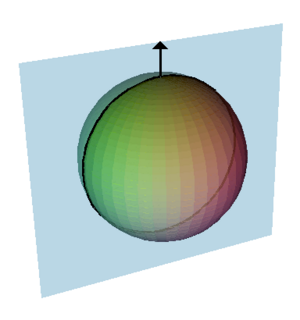A normal vector to a sphere, a normal plane and its normal section. The curvature of the curve of intersection is the sectional curvature. For the sphere each normal section through a given point will be a circle of the same radius, the radius of the sphere. This means that every point on the sphere will be an umbilical point.
7. "All points of a sphere are umbilics."
8. At any point on a surface we can find a normal direction which is at right angles to the surface, for the sphere these on the lines radiating out from the center of the sphere. The intersection of a plane containing the normal with the surface will form a curve called a "normal section" and the curvature of this curve is the "sectional curvature." For most points on a surfaces different sections will have different curvatures, the maximum and minimum values of these are called the principal curvatures. It can be proved that any closed surface will have at least four points called "umbilical points." At an umbilic, all the sectional curvatures are equal, in particular the principal curvature's are equal. Umbilical points can be thought of as the points where the surface is closely approximated by a sphere.
9. For the sphere the curvatures of all normal sections are equal, so every point is an umbilic. The sphere and plane are the only surfaces with this property.
10. "The sphere does not have a surface of centers."
11. For a given normal section there is a circle whose curvature is the same as the sectional curvature, is tangent to the surface and whose center lines along on the normal line. Take the two center corresponding to the maximum and minimum sectional curvatures these are called the "focal points," and the set of all such centers forms the focal surface.
12. For most surfaces the focal surface forms two sheets each of which is a surface and which come together at umbilical points. There are a number of special cases. For canal surfaces one sheet forms a curve and the other sheet is a surface; For cones, cylinders, toruses and cyclides both sheets form curves. For the sphere the center of every osculating circle is at the center of the sphere and the focal surface forms a single point. This is a unique property of the sphere.
13. "All geodesics of the sphere are closed curves."
14. Geodesics are curves on a surface which give the shortest distance between two points. They are generalization of the concept of a straight line in the plane. For the sphere the geodesics are great circles. There are many other surfaces with this property.
15. "Of all the solids having a given volume, the sphere is the one with the smallest surface area; of all solids having a given surface area, the sphere is the one having the greatest volume."
16. These properties define the sphere uniquely. These properties can be seen by observing soap bubbles. A soap bubble will enclose a fixed volume and, due to surface tension, it will try to minimize its surface area. Therefore a free floating soap bubble will be approximately a sphere, factors like gravity will cause a slight distortion.
17. "The sphere has the smallest total mean curvature among all convex solids with a given surface area."
18. The mean curvature is the average of the two principal curvatures and as these are constant at all points of the sphere then so is the mean curvature.
19. "The sphere has constant positive mean curvature."
20. The sphere is the only surface without boundary or singularities with constant positive mean curvature. There are other surfaces with constant mean curvature, the minimal surfaces have zero mean curvature.
21. "The sphere has constant positive Gaussian curvature."
22. Gaussian curvature is the product of the two principle curvatures. It is an intrinsic property which can be determined by measuring length and angles and does not depend on the way the surface is embedded in space. Hence, bending a surface will not alter the Gaussian curvature and other surfaces with constant positive Gaussian curvature can be obtained by cutting a small slit in the sphere and bending it. All these other surfaces would have boundaries and the sphere is the only surface without boundary with constant positive Gaussian curvature. The pseudosphere is an example of a surface with constant negative Gaussian curvature.
23. "The sphere is transformed into itself by a three-parameter family of rigid motions."
24. Consider a unit sphere place at the origin, a rotation around the x, y, or z axis will map the sphere onto itself, indeed any rotation about a line through the origin can be expressed as a combination of rotations around the three coordinate axis, see Euler angles. Thus there is a three parameter family of rotations which transform the sphere onto itself, this is the rotation group, SO(3). The plane is the only other surface with a three parameter family of transformations (translations along the x and y axis and rotations around the origin). Circular cylinders are the only surfaces with two parameter families of rigid motions and the surfaces of revolution and helicoids are the only surfaces with a one parameter family.